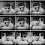# Computed Column in SQL Server

In this article, we will see what is 'Computed Column in SQL Server.

First of all What is a Computed column in SQL Server?

Computed column as the name gives an idea it's related to something calculation/ computation, Yes, computed columns are similar to a column in Excel with an applied formula that calculates the value automatically of the given query or the columns.
Computed columns are columns with some user-defined expression or with some formula to calculate the corresponding columns with operators or with some query.

Let's take an example:

Creating a table named 'CalculationTable' with a computed column named 'Age'

 Create table CalculationTable        (               ID INT IDENTITY(1,1) PRIMARY KEY,               NAME NVARCHAR(50) null,               DATEOFBIRTH DATE null,               AGE AS (DATEDIFF(YEAR,DATEOFBIRTH,GETDATE()))        )

You can also use the SSMS user interface to define that column (formula), Let's see how:

Now let's insert some data into the table:

In the above example, AGE  is a computed column, whenever we insert the value of DATEOFBIRTH  then AGE  will be calculated automatically.

I hope this will be helpful to understand the Computed Columns in SQL Server.

1.1.Thanks!! and keep exploring : https://www.dotnetkida.com

2.1.Thanks!!! and keep visiting : https://www.dotnetkida.com

3.Nice excellent

1.Thanks!!! and don't forget to subscribe us
https://www.dotnetkida.com

2.